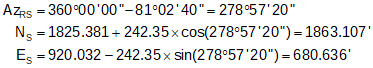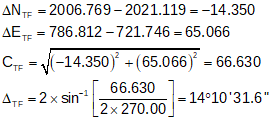### b. Curvilinear Traverse

Lets add a lot and compute its coordinates and area.Starting at point C, compute points Q, R, and S. Convert bearings to azimuhts for ease of computations.Point T must be computed by intersecting the westerly lot line with the southerly street sideline. Point T is connected to point S by a bearing and point O by the southerly curve radius.Line SO will serve as the baseline for the intersection triangle. Compute its length and direction from point S's and O's coordinates.Compute angle at S from directions of SO and ST:Use Law of Sines to solve angle at T:(since the angle at T is less than 90°, this is the correct angle). Compute angle at O:And length of line ST:Finally, compute point T from point S:To determine the Lot area, we will use the coordinate method then take into account the segment areas caused by the two arcs. To compute the segment areas we need the central angle for each arc.

 The following equations come from the VII COGO topic. Segment area:(Eqn F-7) Central angle:(Eqn F-3)

Coordinate method area:Arc TF:Arc FQTotal Lot area:Final Lot dimensions: